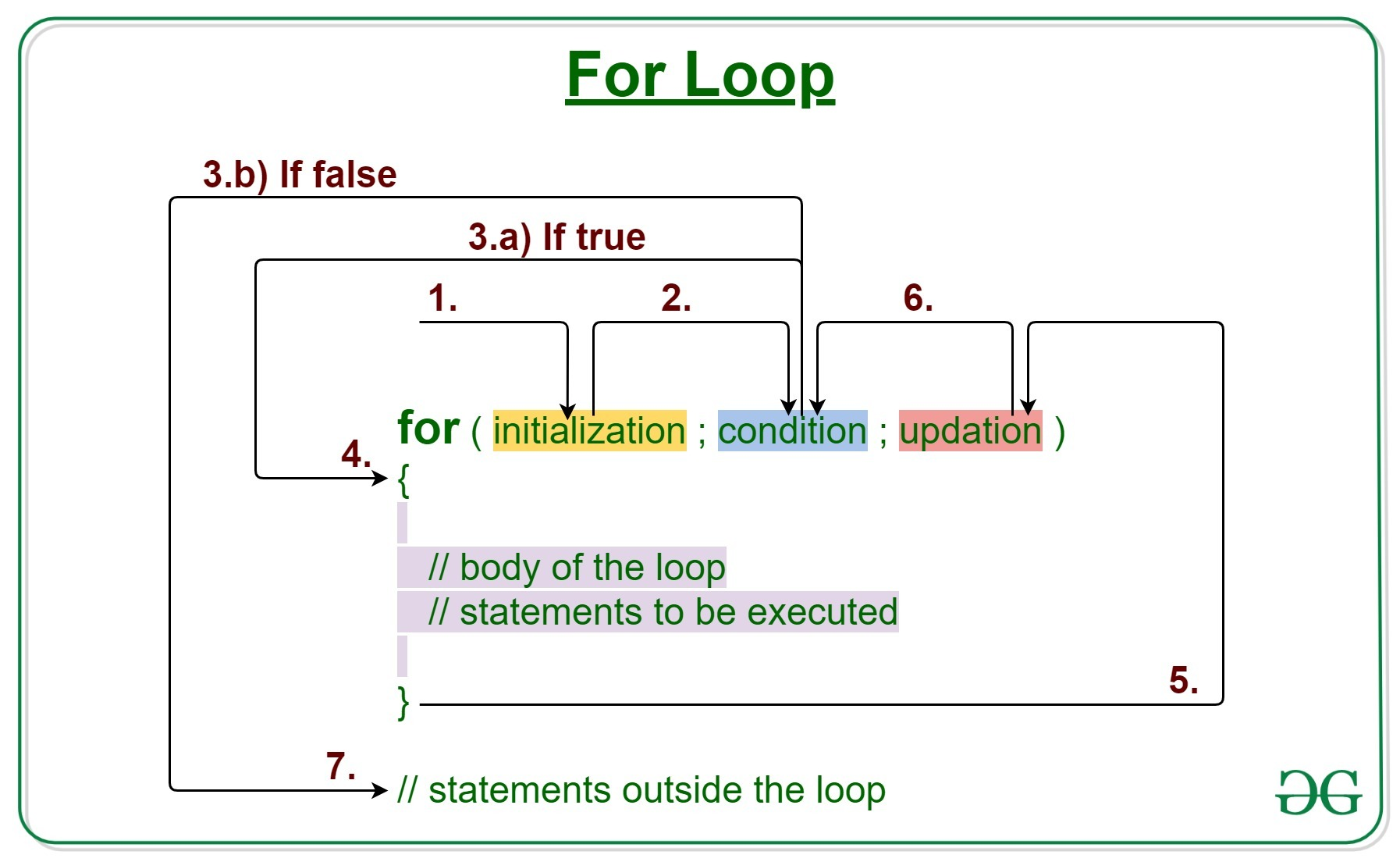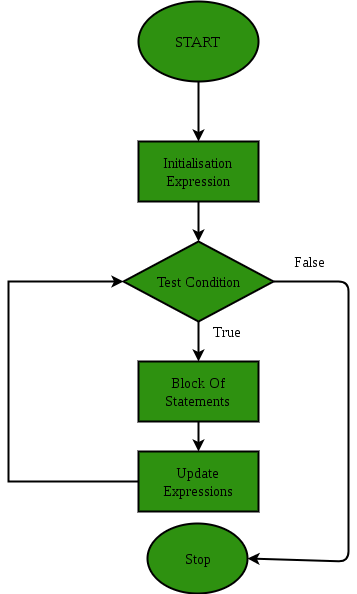Related Articles

# C/C++ For loop with Examples

• Difficulty Level : Basic
• Last Updated : 22 Nov, 2019

Loops in C/C++ come into use when we need to repeatedly execute a block of statements.

For loop is a repetition control structure which allows us to write a loop that is executed a specific number of times. The loop enables us to perform n number of steps together in one line.Syntax:

```for (initialization expr; test expr; update expr)
{
// body of the loop
// statements we want to execute
}
```

The various parts of the For loop are:

1. Initialization Expression: In this expression we have to initialize the loop counter to some value.
Example:
`int i=1;`
2. Condition: In this expression we have to test the condition. If the condition evaluates to true then we will execute the body of the loop and go to update expression. Otherwise, we will exit from the for loop.
Example:
`i <= 10`
3. Update Expression: After executing the loop body, this expression increments/decrements the loop variable by some value.
Example:
`i++;`

How does a For loop executes?

1. Control falls into the for loop. Initialization is done
2. The flow jumps to Condition
3. Condition is tested.
1. If Condition yields true, the flow goes into the Body
2. If Condition yields false, the flow goes outside the loop
4. The statements inside the body of the loop get executed.
5. The flow goes to the Updation
6. Updation takes place and the flow goes to Step 3 again
7. The for loop has ended and the flow has gone outside.

Flow chart for loop (For Control Flow):Example 1: This program will try to print “Hello World” 5 times. The program will execute in the following manner:

## C

 `// C program to illustrate for loop`` ` `#include `` ` `int` `main()``{``    ``int` `i = 0;`` ` `    ``// Writing a for loop``    ``// to print Hello World 5 times``    ``for` `(i = 1; i <= 5; i++) {``        ``printf``(``"Hello World\n"``);``    ``}`` ` `    ``return` `0;``}`

## C++

 `// C++ program to illustrate for loop`` ` `#include ``using` `namespace` `std;`` ` `int` `main()``{`` ` `    ``// Writing a for loop``    ``// to print Hello World 5 times``    ``for` `(``int` `i = 1; i <= 5; i++) {``        ``cout << ``"Hello World\n"``;``    ``}`` ` `    ``return` `0;``}`
Output:
```Hello World
Hello World
Hello World
Hello World
Hello World
```

Dry-Running Example 1:

```1. Program starts.
2. i is initialized with value 1.
3. Condition is checked. 1 <= 5 yields true.
3.a) "Hello World" gets printed 1st time.
3.b) Updation is done. Now i = 2.
4. Condition is checked. 2 <= 5 yields true.
4.a) "Hello World" gets printed 2nd time.
4.b) Updation is done. Now i = 3.
5. Condition is checked. 3 <= 5 yields true.
5.a) "Hello World" gets printed 3rd time
5.b) Updation is done. Now i = 4.
6. Condition is checked. 4 <= 5 yields true.
6.a) "Hello World" gets printed 4th time
6.b) Updation is done. Now i = 5.
7. Condition is checked. 5 <= 5 yields true.
7.a) "Hello World" gets printed 5th time
7.b) Updation is done. Now i = 6.
8. Condition is checked. 6 <= 5 yields false.
9. Flow goes outside the loop to return 0
```

Example 2:

## C

 `// C program to illustrate for loop`` ` `#include `` ` `int` `main()``{``    ``int` `i = 0;`` ` `    ``// Writing a for loop``    ``// to print odd numbers upto N``    ``for` `(i = 1; i <= 10; i += 2) {``        ``printf``(``"%d\n"``, i);``    ``}`` ` `    ``return` `0;``}`

## C++

 `// C++ program to illustrate for loop`` ` `#include ``using` `namespace` `std;`` ` `int` `main()``{``    ``int` `i = 0;`` ` `    ``// Writing a for loop``    ``// to print odd numbers upto N``    ``for` `(i = 1; i <= 10; i += 2) {``        ``cout << i << ``"\n"``;``    ``}`` ` `    ``return` `0;``}`
Output:
```1
3
5
7
9
```

Related Articles:

Attention reader! Don’t stop learning now. Participate in the Scholorship Test for First-Step-to-DSA Course for Class 9 to 12 students.

My Personal Notes arrow_drop_up